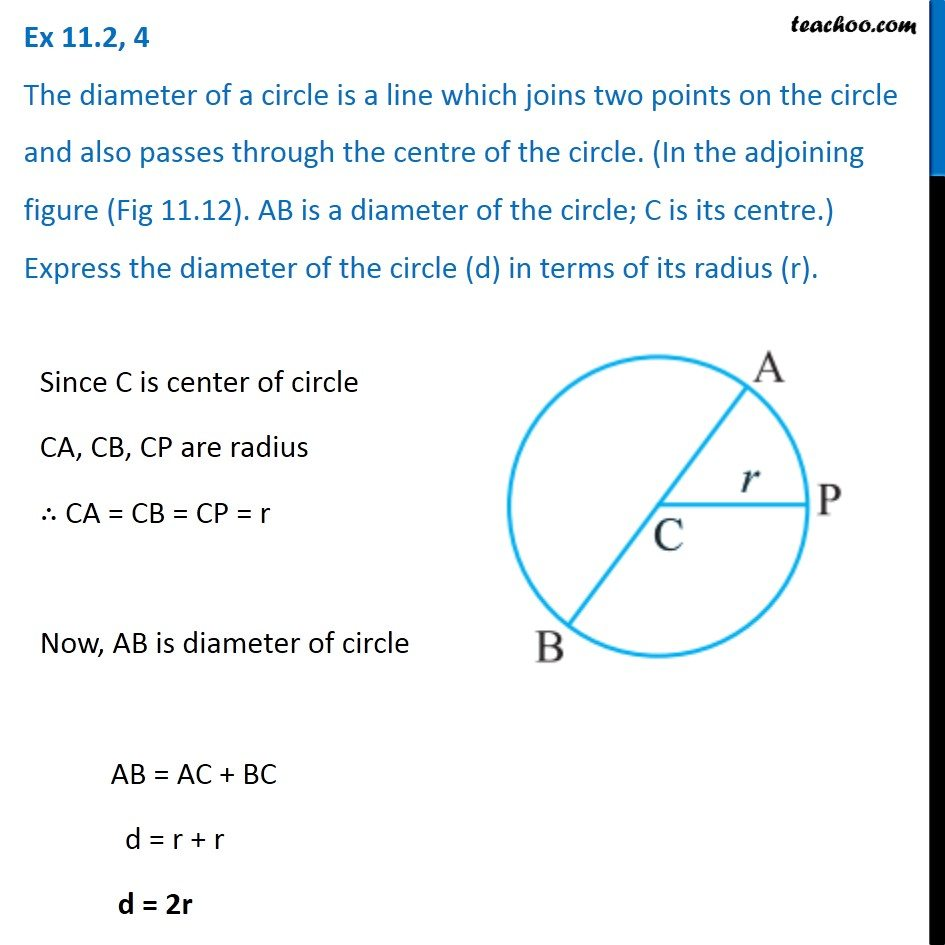Perimeter as variable

Chapter 11 Class 6 Algebra
Concept wiseLearn in your speed, with individual attention - Teachoo Maths 1-on-1 Class

### Transcript

Question 4 The diameter of a circle is a line which joins two points on the circle and also passes through the centre of the circle. (In the adjoining figure (Fig 11.12). AB is a diameter of the circle; C is its centre.) Express the diameter of the circle (d) in terms of its radius (r).Since C is center of circle CA, CB, CP are radius ∴ CA = CB = CP = r Now, AB is diameter of circle AB = AC + BC d = r + r d = 2r